GeeksforGeeks App
Open AppBrowser
Continue

# Complete Roadmap To Learn DSA From Scratch

Today’s world is highly reliable on data and their appropriate management through widely used apps and software. The backbone for appropriate management of data is Data Structure and Algorithms (for convenience here we will use the term DSA). It is a dream for many to achieve expertise in handling and creating these apps and software. With this target in mind, they set out on the journey of learning DSA. The very first step in the journey is the creation of a complete roadmap to learn data structure and algorithms.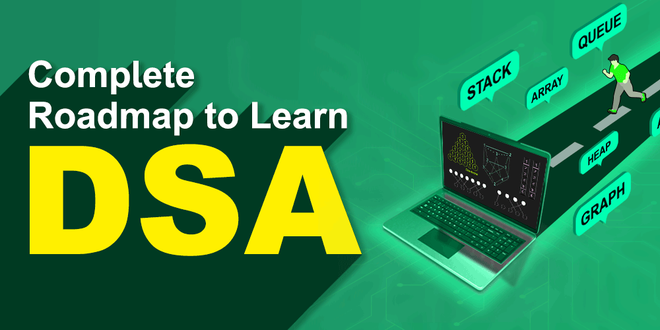Complete Roadmap to Learn Data Structure and Algorithms

Here in this article, we will try to make that task easy for you. We will be providing here with a complete roadmap for learning data structure and algorithms for anyone keen to learn DSA, from scratch.

DSA – Self Paced Course

From creating Games to building Social Media Algorithms. DSA plays an integral part whether you want to build something of your own or either may be willing to get a job in big tech giants like Google, Microsoft, Netflix and more. This time, learn DSA with us, with our most popular DSA course, trusted by over 75,000 students! Designed by leading experts having years of industry expertise, which gives you a complete package of video lectures, practice problems, quizzes, and contests. Get started!

## 5 Steps to learn DSA from scratch

The first and foremost thing is dividing the total procedure into little pieces which need to be done sequentially.

The complete process to learn DSA from scratch can be broken into 5 parts:

1. Learn a programming language of your choice
2. Learn about Time and Space complexities
3. Learn the basics of individual Data Structures and Algorithms
4. Practice, Practice, and Practice more
5. Compete and Become a Pro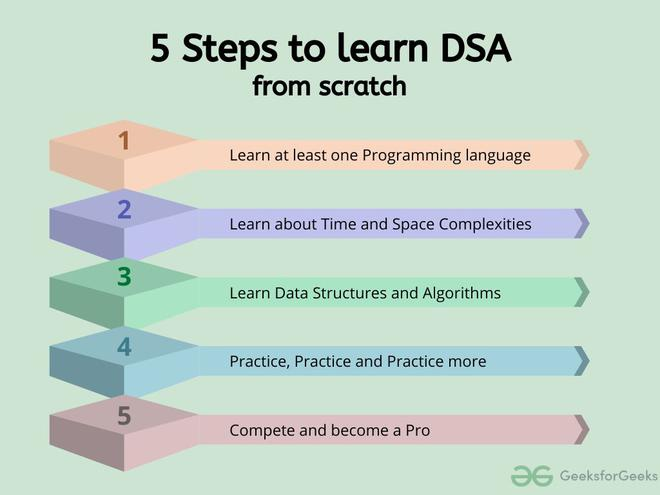5 Steps to learn DSA from scratch

Before starting any data structure or algorithm you need to know the means to express it or implement it. So, the first task is to learn any programming language. Then you should learn about one of the most important and most used concepts about DSA, the complexity of a program. Now equipped with the prerequisites, you can start learning DSA and at the same time practice it regularly and compete in challenges to gauge and sharpen your ability. In the following sections, we will discuss each of the steps in detail.

## 1. Learn at least one Programming language

This should be your first step while starting to learn data structure and algorithms. We as human beings, before learning to write a sentence or an essay on a topic, first try to learn that language: the alphabet, letters, and punctuations in it, how and when to use them. The same goes for programming also.

Firstly, select a language of your choice, be it Java, C, C++, Python, or any other language of your choice. Before learning how to code in that language you should learn about the building pieces of the language: the basic syntax, the data types, variables, operators, conditional statements, loops, functions, etc. You may also learn the concept of OOP (Object Oriented Programming)

To help you get started with the language of your choice, we have created a complete course to start as a beginner, such as:

You can also explore our other courses for Programming languages on our Practice portal.

Here comes one of the interesting and important topics. The primary motive to use DSA is to solve a problem effectively and efficiently. How can you decide if a program written by you is efficient or not? This is measured by complexities. Complexity is of two types:

1. Time Complexity: Time complexity is used to measure the amount of time required to execute the code.
2. Space Complexity: Space complexity means the amount of space required to execute successfully the functionalities of the code.
You will also come across the term Auxiliary Space very commonly in DSA, which refers to the extra space used in the program other than the input data structure.

Both of the above complexities are measured with respect to the input parameters. But here arises a problem. The time required for executing a code depends on several factors, such as:

• The number of operations performed in the program,
• The speed of the device, and also
• The speed of data transfer if being executed on an online platform.

So how can we determine which one is efficient? The answer is the use of asymptotic notation.

Asymptotic notation is a mathematical tool that calculates the required time in terms of input size and does not require the execution of the code.

It neglects the system-dependent constants and is related to only the number of modular operations being performed in the whole program. The following 3 asymptotic notations are mostly used to represent the time complexity of algorithms:

• Big-O Notation (Ο) – Big-O notation specifically describes the worst-case scenario.
• Omega Notation (Ω) – Omega(Ω) notation specifically describes the best-case scenario.
• Theta Notation (θ) – This notation represents the average complexity of an algorithm.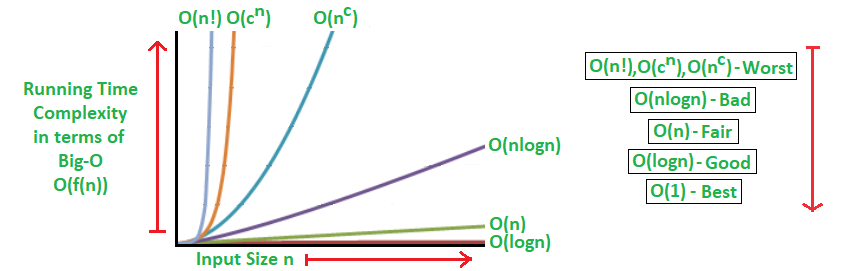Rate of Growth of Algorithms

The most used notation in the analysis of a code is the Big O Notation which gives an upper bound of the running time of the code (or the amount of memory used in terms of input size).

To learn about complexity analysis in detail, you can refer to our complete set of articles on the Analysis of Algorithms.

## 3. Learn Data Structures and Algorithms

Here comes the most crucial and the most awaited stage of the roadmap for learning data structure and algorithm – the stage where you start learning about DSA. The topic of DSA consists of two parts:

• Data Structures
• Algorithms

Though they are two different things, they are highly interrelated, and it is very important to follow the right track to learn them most efficiently. If you are confused about which one to learn first, we recommend you to go through our detailed analysis on the topic: What should I learn first- Data Structures or Algorithms?

Here we have followed the flow of learning a data structure and then the most related and important algorithms used by that data structure.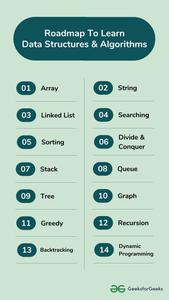### 3.1. Array

The most basic yet important data structure is the array. It is a linear data structure. An array is a collection of homogeneous data types where the elements are allocated contiguous memory. Because of the contiguous allocation of memory, any element of an array can be accessed in constant time. Each array element has a corresponding index number.

Here are some topics about array which you must learn:

• Rotation of Array – Rotation of array means shifting the elements of an array in a circular manner i.e., in the case of right circular shift the last element becomes the first element, and all other element moves one point to the right.
• Rearranging an array – Rearrangement of array elements suggests the changing of an initial order of elements following some conditions or operations.
• Range queries in the array – Often you need to perform operations on a range of elements. These functions are known as range queries.
• Multidimensional array – These are arrays having more than one dimension. The most used one is the 2-dimensional array, commonly known as a matrix.
• Dutch national flag algorithm

### 3.2. String

A string is also a type of array. It can be interpreted as an array of characters. But it has some special characteristics like the last character of a string is a null character to denote the end of the string. Also, there are some unique operations, like concatenation which concatenates two strings into one.

Here we are providing you with some must-know concepts of string:

• Subsequence and substring – A subsequence is a sequence that can be derived from a string deleting one or more elements. A substring is a contiguous segment of the string.
• Reverse and rotation in a string – Reverse operation is interchanging the position of characters of a string such that the first becomes the last, the second becomes the second last, and so on.
• Binary String – A binary string is a string made up of only two types of characters.
• Palindrome – A palindrome string is a string in which the elements at the same distance from the center of the string are the same.
• Lexicographic pattern – Lexicographical pattern is the pattern based on the ASCII value or can be said in dictionary order.
• Pattern searching – Pattern searching is searching a given pattern in the string. It is an advanced topic of string.

The topics which you must want to cover are:

• Singly Linked List – In this, each node of the linked list points only to its next node.
• Circular Linked List – This is the type of linked list where the last node points back to the head of the linked list.
• Doubly Linked List – In this case, each node of the linked list holds two pointers, one point to the next node and the other points to the previous node.

### 3.4. Searching Algorithm

Now we have learned about some linear data structures and is time to learn about some basic and most used algorithms which are hugely used in these types of data structures. One such algorithm is the searching algorithm.

Searching algorithms are used to find a specific element in an array, string, linked list, or some other data structure.

The most common searching algorithms are:

• Linear Search – In this searching algorithm, we check for the element iteratively from one end to the other.
• Binary Search – In this type of searching algorithm, we break the data structure into two equal parts and try to decide in which half we need to find for the element.
• Ternary Search – In this case, the array is divided into three parts, and based on the values at partitioning positions we decide the segment where we need to find the required element.

Besides these, there are other searching algorithms also like

### 3.5. Sorting Algorithm

Here is one other most used algorithm. Often we need to arrange or sort data as per a specific condition. The sorting algorithm is the one that is used in these cases. Based on conditions we can sort a set of homogeneous data in order like sorting an array in increasing or decreasing order.

Sorting Algorithm is used to rearrange a given array or list elements according to a comparison operator on the elements. The comparison operator is used to decide the new order of element in the respective data structure.An example to show Sorting

There are a lot of different types of sorting algorithms. Some widely used algorithms are:

There are several other sorting algorithms also and they are beneficial in different cases. You can learn about them and more in our dedicated article on Sorting algorithms.

### 3.6. Divide and Conquer Algorithm

This is one interesting and important algorithm to be learned in your path of programming. As the name suggests, it breaks the problem into parts, then solves each part and after that again merges the solved subtasks to get the actual problem solved.

Divide and Conquer is an algorithmic paradigm. A typical Divide and Conquer algorithm solves a problem using following three steps.

1. Divide: Break the given problem into subproblems of same type.
2. Conquer: Recursively solve these subproblems
3. Combine: Appropriately combine the answers

This is the primary technique mentioned in the two sorting algorithms Merge Sort and Quick Sort which are mentioned earlier. To learn more about the technique, the cases where it is used, and its implementation and solve some interesting problems, please refer to the dedicated article Divide and Conquer Algorithm.

### 3.7. Stack

Now you should move to some more complex data structures, such as Stack and Queue.

Stack is a linear data structure which follows a particular order in which the operations are performed. The order may be LIFO(Last In First Out) or FILO(First In Last Out).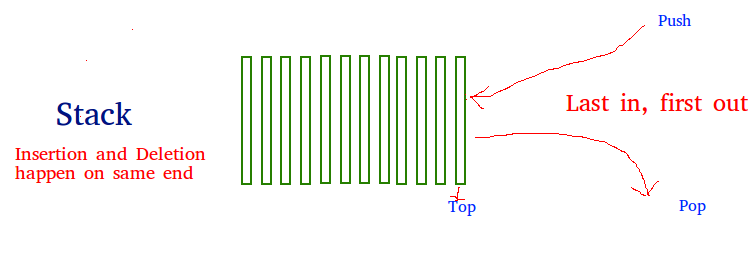Stack Data Structure

The reason why Stack is considered a complex data structure is that it uses other data structures for implementation, such as Arrays, Linked lists, etc. based on the characteristics and features of Stack data structure.

### 3.8. Queue

Another data structure that is similar to Stack, yet different in its characteristics, is Queue.

A Queue is a linear structure which follows First In First Out (FIFO) approach in its individual operations.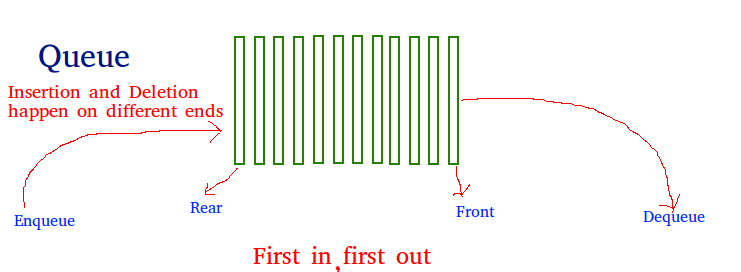Queue Data Structure

A queue can be of different types like

• Circular queue – In a circular queue the last element is connected to the first element of the queue
• Double-ended queue (or known as deque) – A double-ended queue is a special type of queue where one can perform the operations from both ends of the queue.
• Priority queue – It is a special type of queue where the elements are arranged as per their priority. A low priority element is dequeued after a high priority element.

### 3.9. Tree Data Structure

After having the basics covered about the linear data structure, now it is time to take a step forward to learn about the non-linear data structures. The first non-linear data structure you should learn is the tree.

Tree data structure is similar to a tree we see in nature but it is upside down. It also has a root and leaves. The root is the first node of the tree and the leaves are the ones at the bottom-most level. The special characteristic of a tree is that there is only one path to go from any of its nodes to any other node.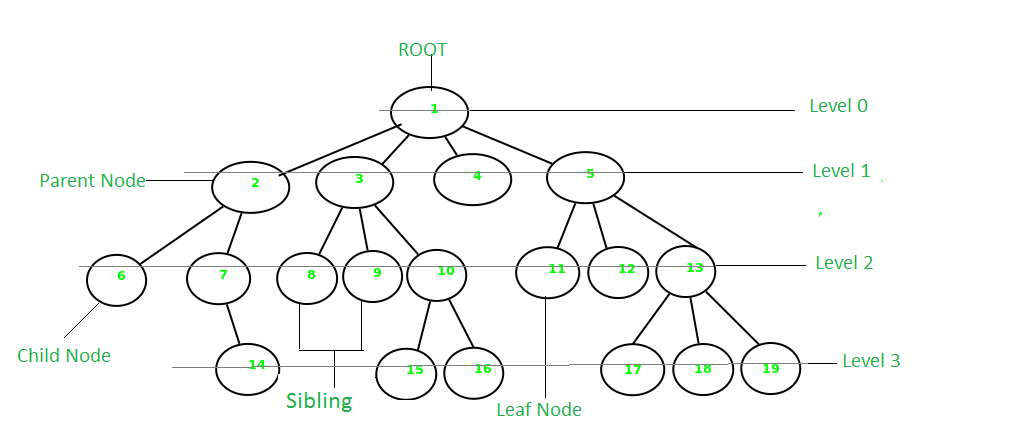Tree Data Structure

Based on the maximum number of children of a node of the tree it can be –

• Binary tree – This is a special type of tree where each node can have a maximum of 2 children.
• Ternary tree – This is a special type of tree where each node can have a maximum of 3 children.
• N-ary tree – In this type of tree, a node can have at most N children.

Based on the configuration of nodes there are also several classifications. Some of them are:

• Complete Binary Tree – In this type of binary tree all the levels are filled except maybe for the last level. But the last level elements are filled as left as possible.
• Perfect Binary Tree – A perfect binary tree has all the levels filled
• Binary Search Tree – A binary search tree is a special type of binary tree where the smaller node is put to the left of a node and a higher value node is put to the right of a node
• Ternary Search Tree – It is similar to a binary search tree, except for the fact that here one element can have at most 3 children.

### 3.10. Graph Data Structure

Another important non-linear data structure is the graph. It is similar to the Tree data structure, with the difference that there is no particular root or leaf node, and it can be traversed in any order.

A Graph is a non-linear data structure consisting of a finite set of vertices(or nodes) and a set of edges that connect a pair of nodes.Graph Data Structure

Each edge shows a connection between a pair of nodes. This data structure helps solve many real-life problems. Based on the orientation of the edges and the nodes there are various types of graphs.

Here are some must to know concepts of graphs:

### 3.11. Greedy methodology

As the name suggests, this algorithm builds up the solution one piece at a time and chooses the next piece which gives the most obvious and immediate benefit i.e., which is the most optimal choice at that moment. So the problems where choosing locally optimal also leads to the global solutions are best fit for Greedy.

For example, consider the Fractional Knapsack Problem. The local optimal strategy is to choose the item that has maximum value vs weight ratio. This strategy also leads to a globally optimal solution because we are allowed to take fractions of an item.Fractional Knapsack Problem

Here is how you can get started with the Greedy algorithm with the help of relevant sub-topics:

### 3.12. Recursion

Recursion is one of the most important algorithms which uses the concept of code reusability and repeated usage of the same piece of code.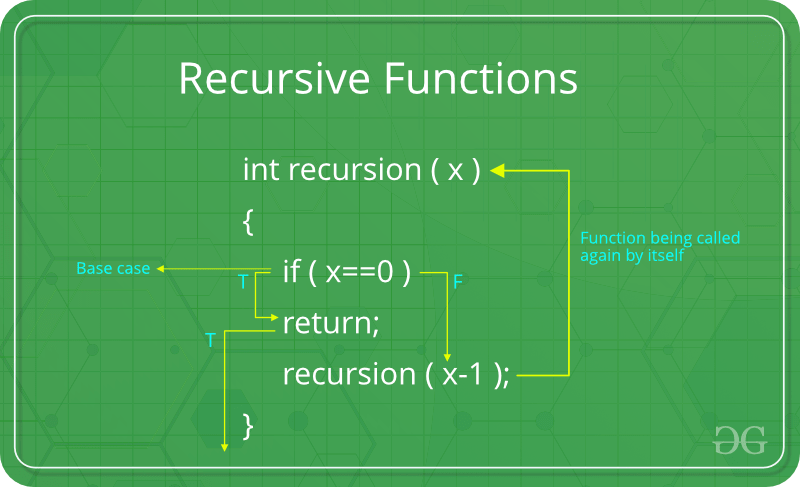Recursion

The point which makes Recursion one of the most used algorithms is that it forms the base for many other algorithms such as:

In Recursion, you can follow the below articles/links to get the most out of it:

### 3.13. Backtracking Algorithm

As mentioned earlier, the Backtracking algorithm is derived from the Recursion algorithm, with the option to revert if a recursive solution fails, i.e. in case a solution fails, the program traces back to the moment where it failed and builds on another solution. So basically it tries out all the possible solutions and finds the correct one.

Backtracking is an algorithmic technique for solving problems recursively by trying to build a solution incrementally, one piece at a time, removing those solutions that fail to satisfy the constraints of the problem at any point of time

Some important and most common problems of backtracking algorithms, that you must solve before moving ahead, are:

### 3.14. Dynamic Programming

Another crucial algorithm is dynamic programming. Dynamic Programming is mainly an optimization over plain recursion. Wherever we see a recursive solution that has repeated calls for the same inputs, we can optimize it using Dynamic Programming.

The main concept of the Dynamic Programming algorithm is to use the previously calculated result to avoid repeated calculations of the same subtask which helps in reducing the time complexity.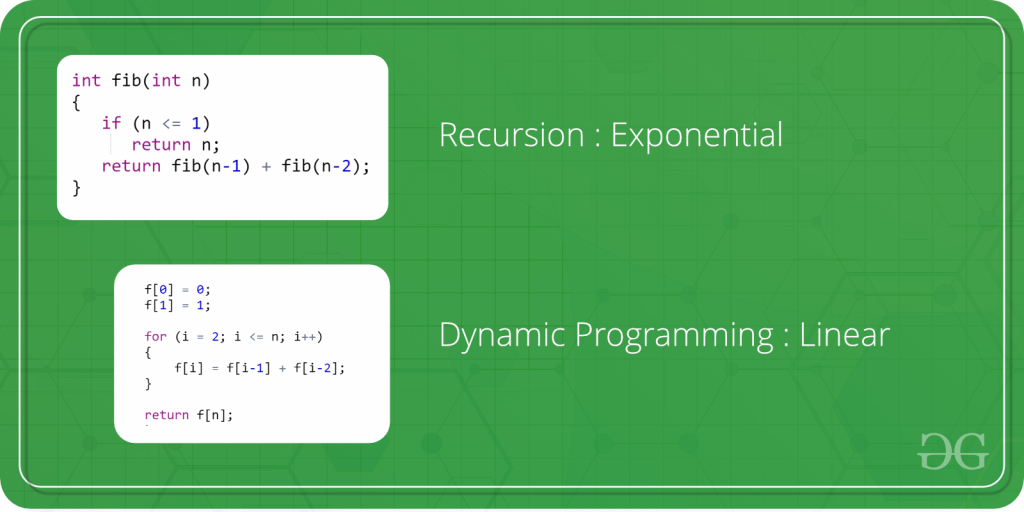Dynamic Programming

To learn more about dynamic programming and practice some interesting problems related to it, refer to the following articles:

## 4. Practice, Practice and Practice more

With this, we have completed the basics of major Data structure and Algorithms, and now it’s time to try our hands on each of them.

This is highly applicable for learning DSA. You have learned a lot of data structures and algorithms and now you need a lot of practice. This may be seen as a separate step or an integrated part of the process of learning DSA. Because of its importance, we are discussing it as a separate step.

For practicing problems on individual data structures and algorithms, you can use the following links:

Apart from these, there are many other practice problems that you can refer based on their respective difficulties:

You can also try to solve the most asked interview questions based on the list curated by us at:

You can also try our curated lists of problems from below articles:

## 5. Compete and Become A Pro

Now it is time to test out your skills and efficiency. The best possible way is to compete with others. This will help you find out your position among others and also give you a hint on the areas you are lacking.

There are several online competitive platforms available where you can participate regularly. Also, some online challenges are held from time to time in a year which also provides lots of prizes and opportunities, such as:

• Monthly Job-a-thon: It is a contest for individual participants. Participants get the opportunity to get hired by a bunch of companies that shortlist for interviews as per their criteria.
• Bi-Wizard Coding: A coding competition exclusively for students. The top 100 students get chances of winning exciting rewards and also access to free courses.
• Interview Series: A weekly challenge that gives a great opportunity for aspirants to practice a lot of questions based on important data structure and algorithms concepts for the preparation of interviews.
• Problem of the Day: A new problem every day to strengthen the base of data structure and algorithm.

To learn more about where to compete, you can refer to our detailed article Top 15 Websites for Coding Challenges and Competitions.

## Tips to boost your learning

By far we have discussed in-depth the 5 crucial steps to learning DSA from scratch. During the complete journey on the roadmap to learn DSA, here are some tips which will surely help you:

### Learn the Fundamentals of chosen Programming Language thoroughly

Implement each small concept that you are learning. Make sure to learn the following concepts:

• Basic Syntax
• Data Types
• Operators, Variables, functions
• Conditional Statement, loops
• OOP(Object-Oriented Programming)

### Get a good grasp of the Complexity Analysis

Understand how the complexity is calculated, and try to solve multiple questions to find the complexities of programs. You can also try out our quiz on Analysis of Algorithms for better practice.

### Focus on Logic Building

The best way to do this is to solve as many problems as you can from scratch, without looking into solutions or editorials. The more you solve, the more strong your logic building will be.

### Stuck on a problem/topic? Don’t worry, you are not alone

It is a brainer that you can solve all the problems all by yourself.

There will be problems, hours, and even days when you will be stuck and won’t be able to find any solution.

Don’t worry, it happens with everyone. If stuck on any problem try to read hints and approaches for solutions. If still unable then see the logic only and code it on your own. If getting stuck on similar types of problems you should probably revise the concept before trying to solve similar types of problems again.

You can also try out our 24×7 Doubt Assistance program to let us help you tackle such situations without breaking a sweat.

### Be consistent

Every monument is built brick by brick by working daily, consistently, and so is the case for DSA. You must try to learn at least 1 new topic every day and solve at least 1 new problem related to it every day. Making this a practice for each day every day will help you master DSA in the best possible manner.

Make sure to give coding challenges at regular intervals as well. You might face challenges in solving even 1 problem in the beginning, but in the end, it will be all worth it. You can try GeeksforGeeks POTD to solve one problem based on DSA every day and here you can also use the discussion forums to help you make sure you get the logic properly. To know more about the discussion portals read the article – Stuck in Programming: Get The Solution From These 10 Best Websites.

## Conclusion

Is that it? Is this all required to master Data Structures and Algorithms and become Hero from Zero in DSA? Well if you have gone through the above-mentioned roadmap for learning DSA, then this is it. You have successfully started, learned, practiced, and competed enough to call yourself a DSA Pro.

But, like the universe, learning is endless. You can never learn everything about any topic. So make sure to keep practicing and keep updating yourself with new competitions, topics, and problems.

Related articles:

My Personal Notes arrow_drop_up